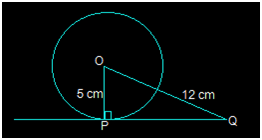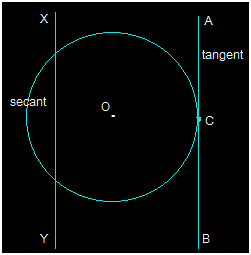• +91 9971497814
• info@interviewmaterial.com

# Circles Ex-10.1 Interview Questions Answers

### Related Subjects

Question 1 :

How many tangentscan a circle have?

There can be infinite tangents to a circle. A circle is made up ofinfinite points which are at an equal distance from a point. Since there areinfinite points on the circumference of a circle, infinite tangents can bedrawn from them.

Question 2 :

Fill in the blanks:

(i) A tangent to a circleintersects it in …………… point(s).

(ii) A line intersecting a circle in two points is called a ………….

(iii) A circle can have …………… parallel tangents at the most.

(iv) The common point of a tangent to a circle and the circle is called …………

(i) A tangent to a circle intersects itin one point(s).

(ii) A line intersecting a circle in twopoints is called a secant.

(iii) A circle can have two parallel tangents at themost.

(iv) The common point of a tangent to a circleand the circle is called the pointof contact.

Question 3 : A tangent PQ at a point P of a circle of radius 5 cm meets a line through the centre O at

a point Q so that OQ= 12 cm. Length PQ is :

(A) 12 cm                      (B) 13 cm

(C) 8.5 cm                     (D) √119 cmIn the above figure, the line that is drawnfrom the centre of the given circle to the tangent PQ is perpendicular to PQ.

And so, OP PQ

Using Pythagoras theorem in triangle ΔOPQ weget,

OQ2 = OP2+PQ2

(12)= 52+PQ2

PQ2 = 144-25

PQ2 = 119

PQ = √119 cm

So, option D i.e. √119 cm is the length of PQ.

Question 4 :

Draw a circle andtwo lines parallel to a given line such that one is a tangent and the

other, a secant tothe circle.

Answer 4 :In the above figure, XY and AB are two theparallel lines. The line segment AB is the tangent at point C while the linesegment XY is the secant.

Todays Deals### Circles Ex-10.1 Contributorskrishan

Name:
Email:

# Latest News# 9000 interview questions in different categories